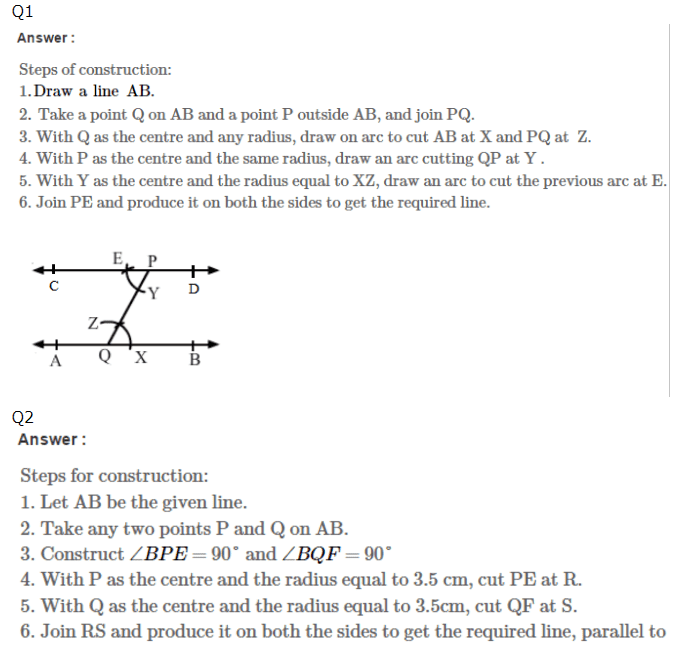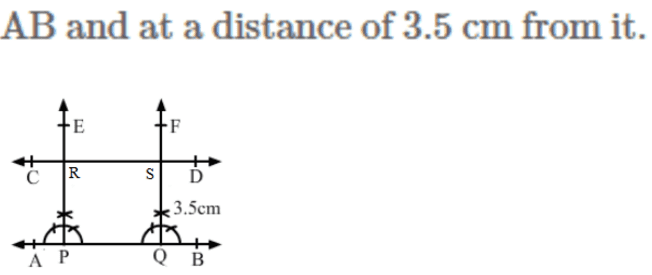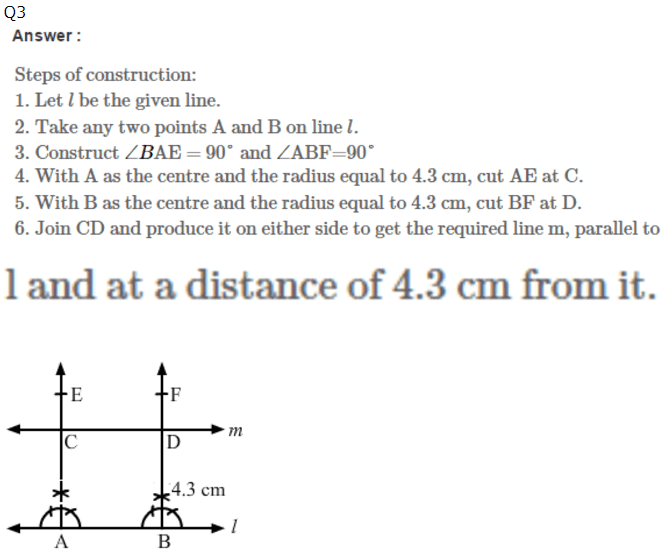RS Aggarwal Class 7 Solutions Chapter 17 - Constructions

RS Aggarwal Class 7 Chapter 17 - Constructions Solutions Free PDF

Constructions is a topic of Geometry, which teaches students the core concepts behind drawing different shapes, lines and angles. Students can use different instruments such as a compass, protractor and a scale for the construction of different geometrical shapes.

Some of the different geometric constructions that students will get to learn in this chapter are:

1. Angle Bisector
2. Line Segment Bisector
3. Pentagon, Hexagon
4. Equilateral Triangle
5. 30, 45, 60, 90, 120 degree angle
6. Center of a circle
7. Tangents on a circle

Check out the RS Aggarwal Class 7 Solutions Chapter 17 Constructions below:Practise This Question

Find the leftmost and the rightmost number when the following numbers are represented on the number line.

1910, 95, 85, 32, 1710